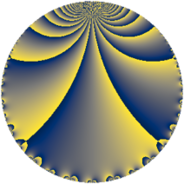# Properties

 Label 32.3.cLevel $32$ Weight $3$ Character orbit 32.c Rep. character $\chi_{32}(31,\cdot)$ Character field $\Q$ Dimension $2$ Newform subspaces $1$ Sturm bound $12$ Trace bound $0$

# Related objects

## Defining parameters

 Level: $$N$$ $$=$$ $$32 = 2^{5}$$ Weight: $$k$$ $$=$$ $$3$$ Character orbit: $$[\chi]$$ $$=$$ 32.c (of order $$2$$ and degree $$1$$) Character conductor: $$\operatorname{cond}(\chi)$$ $$=$$ $$4$$ Character field: $$\Q$$ Newform subspaces: $$1$$ Sturm bound: $$12$$ Trace bound: $$0$$

## Dimensions

The following table gives the dimensions of various subspaces of $$M_{3}(32, [\chi])$$.

Total New Old
Modular forms 12 2 10
Cusp forms 4 2 2
Eisenstein series 8 0 8

## Trace form

 $$2q + 4q^{5} - 14q^{9} + O(q^{10})$$ $$2q + 4q^{5} - 14q^{9} - 28q^{13} + 36q^{17} + 64q^{21} - 42q^{25} - 28q^{29} + 32q^{33} - 60q^{37} - 28q^{41} - 28q^{45} - 30q^{49} + 132q^{53} + 96q^{57} + 164q^{61} - 56q^{65} - 320q^{69} + 132q^{73} - 64q^{77} - 190q^{81} + 72q^{85} - 60q^{89} + 256q^{93} - 28q^{97} + O(q^{100})$$

## Decomposition of $$S_{3}^{\mathrm{new}}(32, [\chi])$$ into newform subspaces

Label Dim. $$A$$ Field CM Traces $q$-expansion
$$a_2$$ $$a_3$$ $$a_5$$ $$a_7$$
32.3.c.a $$2$$ $$0.872$$ $$\Q(\sqrt{-1})$$ None $$0$$ $$0$$ $$4$$ $$0$$ $$q+iq^{3}+2q^{5}-2iq^{7}-7q^{9}-iq^{11}+\cdots$$

## Decomposition of $$S_{3}^{\mathrm{old}}(32, [\chi])$$ into lower level spaces

$$S_{3}^{\mathrm{old}}(32, [\chi]) \cong$$ $$S_{3}^{\mathrm{new}}(16, [\chi])$$$$^{\oplus 2}$$